|

# 吊顶到底该不该吊?怎么吊才好看?

吊顶是很多家庭都会选择的传统装饰，但是随着北欧风极简风的流行，我们不得不承认，繁复的吊顶真的过时了。现在的年轻人好不容易买下一套房子，一方面为了省钱，另一方面为了向极简风靠拢，大多选择不在吊顶上大做文章。要么用石膏线做个边角，要么用墙纸进行装饰，再者用灯具搭配白顶也是不错的选择！这样一来，原本可怜的层高不会变得压抑，可发挥的空间也就更大了。然而，你真的以为那些简单利落的天花造价更低，不用烧钱就能拥有时尚时尚最时尚的吊顶吗？你太天真了！很多看似极简的天花，其实都做了“假吊顶”，而且投入的资金并不低，这就是我们平时所说的简约而不简单。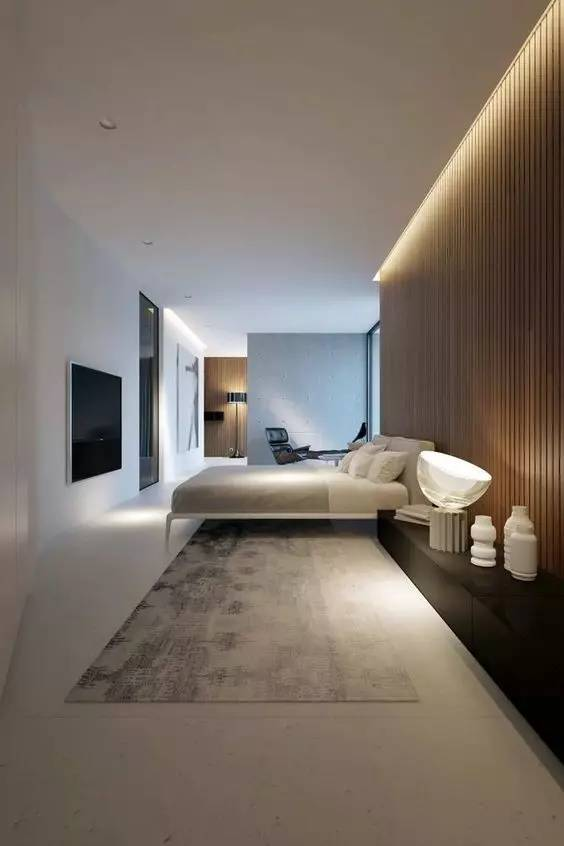那么，到底什么情况下需要做吊顶，什么时候可以省略呢？下面，编辑将从吊顶的功能说起，逐一为大家解答。

吊顶功能

遮盖管道、横梁

有些住宅的横梁或管道露在外面很“煞风景”，吊顶可以起到掩盖和修饰的效果。通过弥补原建筑结构的不足，使顶面整齐有序而不显杂乱。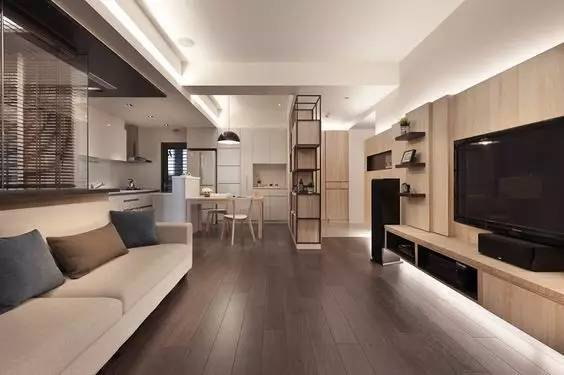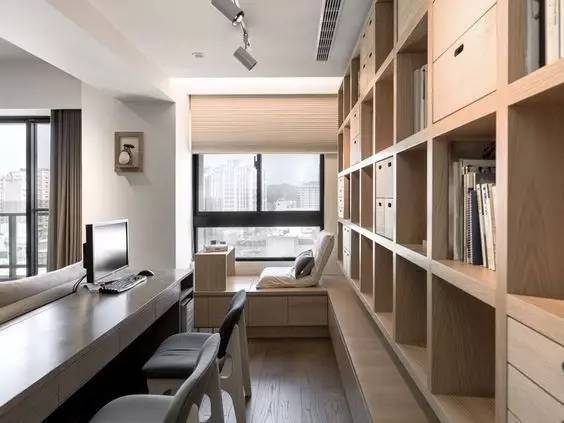另外，当业主想要把阳台的一部分融入客厅，两个区域之间的梁就可以用吊顶来修饰，使顶面处理富有个性，增强视觉感染力。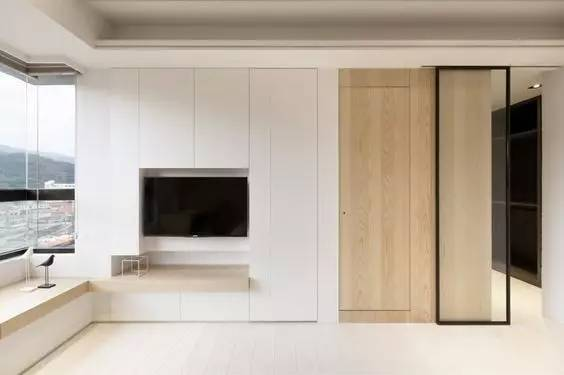空间分区

吊顶是分割空间的隐性手段之一，利用吊顶可以让层高相同的空间营造出功能和视觉上的高低差，从而划分出两个不同的区域。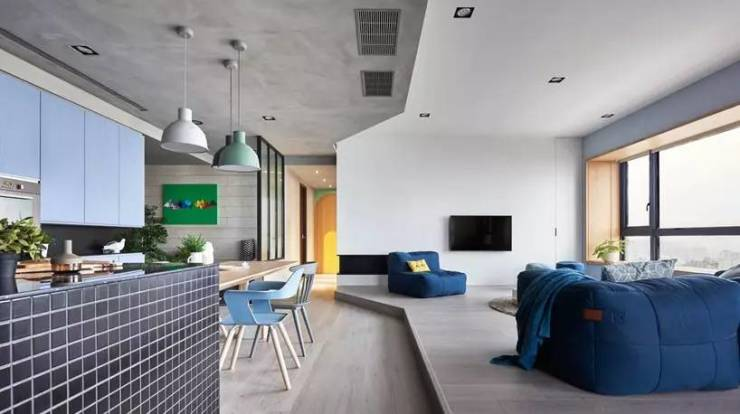例如处于开放式格局中的客厅与餐厅，通过吊顶的分割处理，就可以明确分区，不会导致视觉混淆。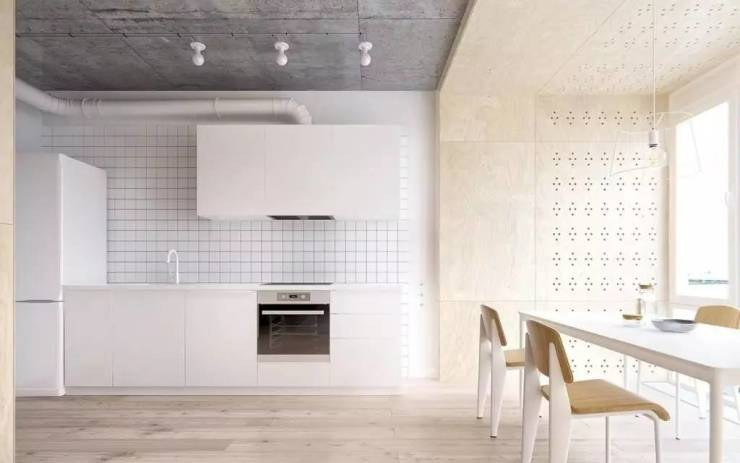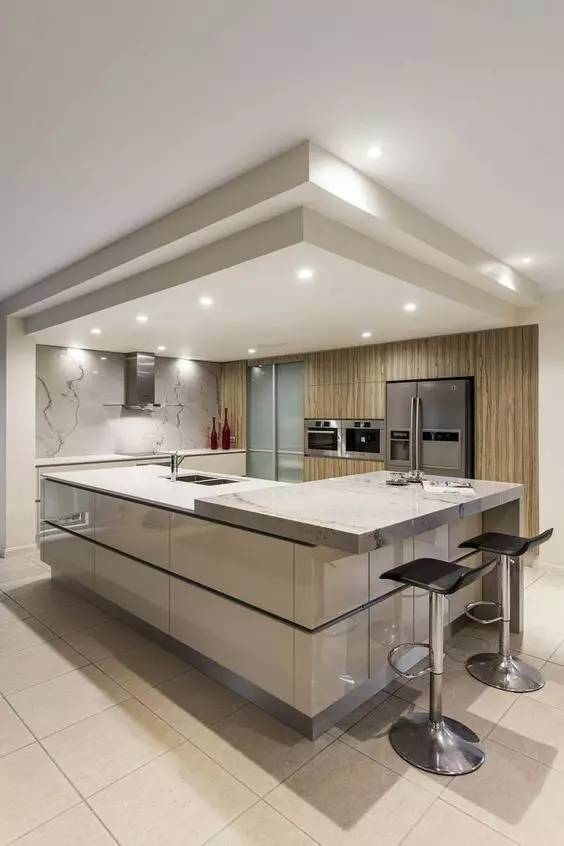当然，在做好吊顶之后，再利用特定的板材来增加辨识度，也是一种备受推崇的手段。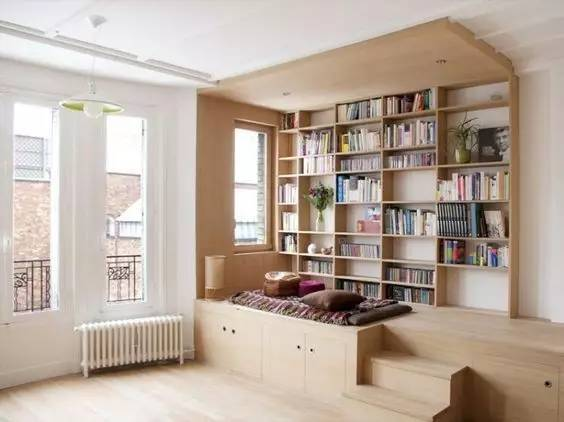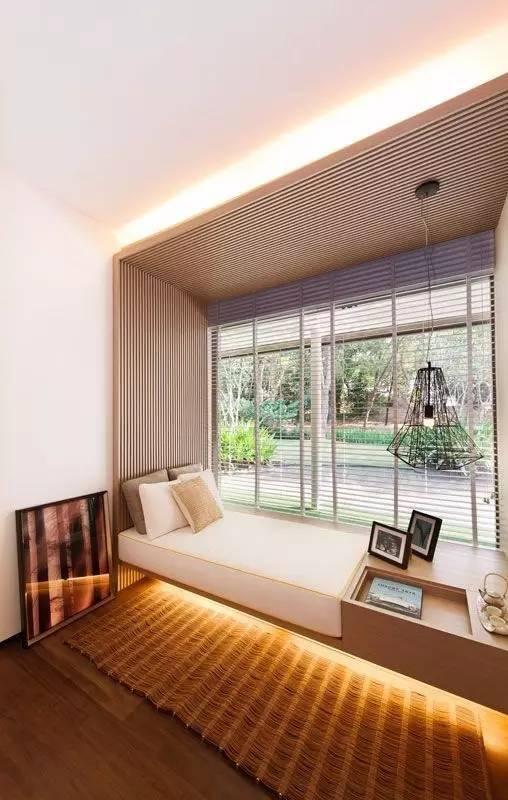隔热保温

吊顶设计不仅要考虑到室内的装饰效果，也要考虑到房屋实际的功能需求。

如顶楼的住宅如无设计隔热层，一到夏天就很可能忍受不了室内温度飙高之苦，通过吊顶加一个隔热层，则会起到隔热降温的作用。而到了冬天，吊顶又可形成一个保温层，使室内的热量不易流失。吊顶的不同种类

（按区域分）

全屋吊顶

全屋吊顶有种模块化快装结构的特点，它承担着居室所有空间吊顶美化的使命。为了减少建材污染对人体的危害，人们大多选择新型环保材料进行装饰，因而装饰效果更好，设计成本也更高。近年来，集成吊顶对比局部吊顶安装速度更快，也更注重美学品位，所以恰到好处地迎合了年轻人的需求，再也不用为跟进木工装修而频繁请假旷工了。局部吊顶

局部吊顶相较于全屋吊顶，涉及的装修面积要小很多，但是工程量并非相应的“大打折扣”。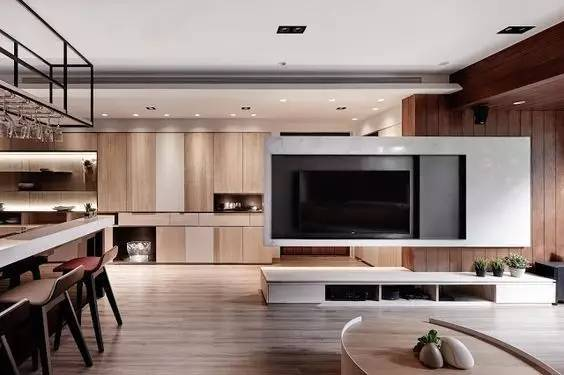因为吊顶的一个重要功能是隐藏管线设备、灯具等，让顶面空间看上去更美观，也省却了繁杂的灯饰。

所以局部吊顶不仅要将许多管线隐藏，还要预留灯具安装部位，使室内产生点光、线光、面光相互辉映的光照效果，对于施工技术也是一项考验。厨卫吊顶

卫生间和厨房的管线非常密集，所以大多数人都会使用吊顶，把这些管线隐藏起来。但在选择材料的时候要更加慎重，最好选择防火、防水、防潮的扣板材料。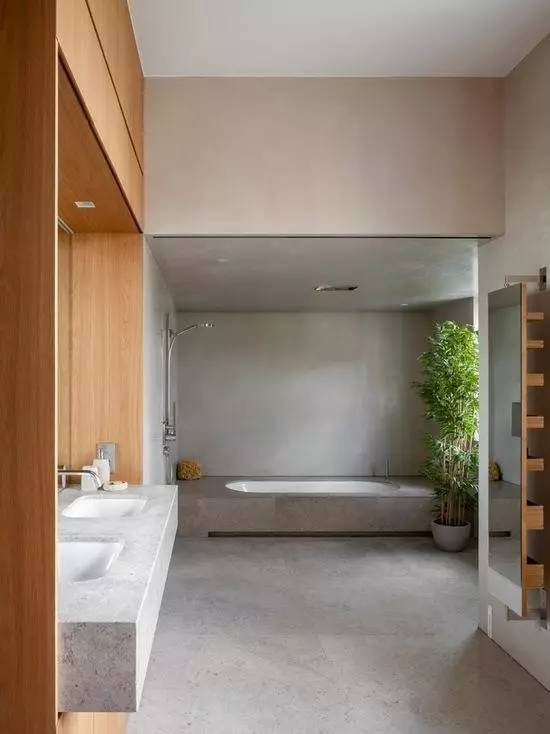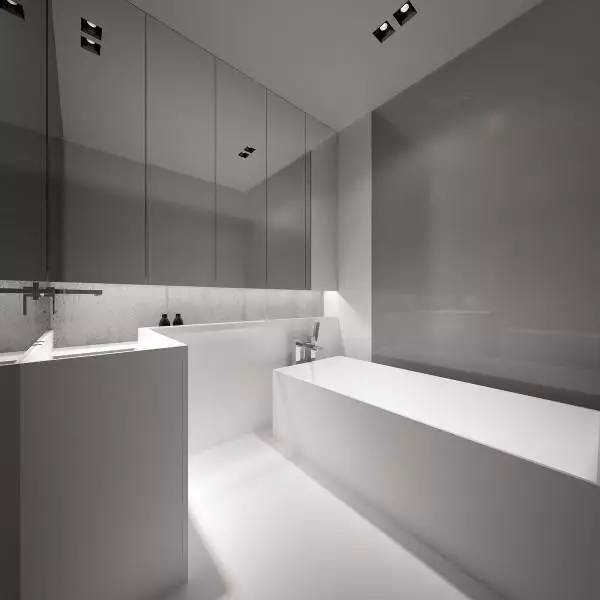（按形式分）

平顶式吊顶

这种形式的吊顶表面上看上去平整简洁，不事雕饰，甚至有一种追求平淡的味道，实际上是用“伪装”来麻痹视觉。它可以用内嵌灯带的形式增加光源，营造独特的室内氛围，对渲染环境、烘托气氛有着重要的作用。回字形吊顶

回字形吊顶是非常常见的吊顶造型。

常用龙骨加石膏板的方式，从天花的四周向内围合，做成四周低中间高的吊顶造型，从而在视觉上形成扩容效果，使房间“变”高。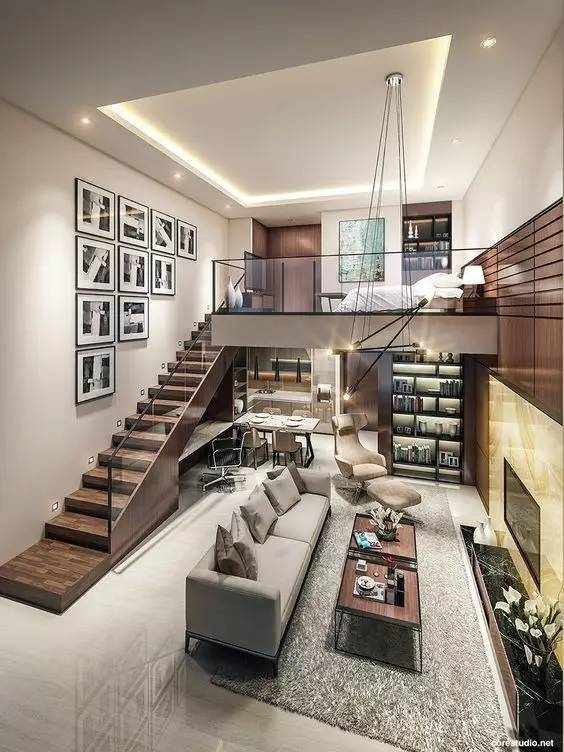吊顶的高度是根据房顶与地面的距离来装修的，如果层高不够的话，最好不要冒险，以免造成难以挽回的后果。异形吊顶

异形吊顶的顶面造型不规则，所以在工艺上的要求是很高的。做得好的话，不仅能让你家的天花无限精彩，还能减少户型的逼仄之感，带来眼前一亮的视觉体验。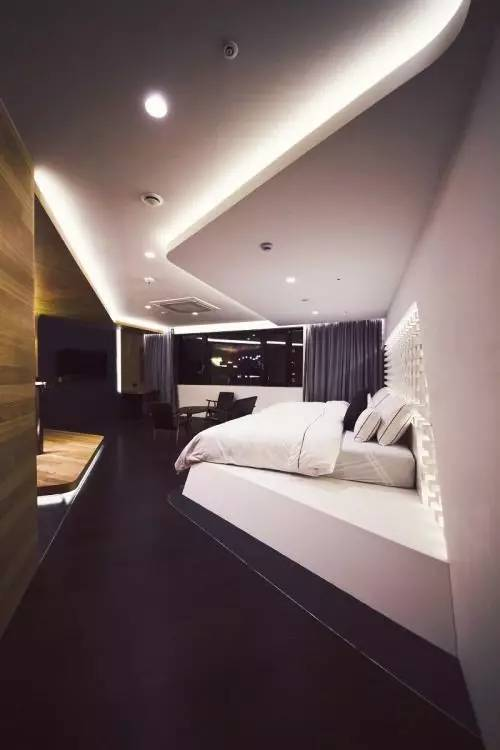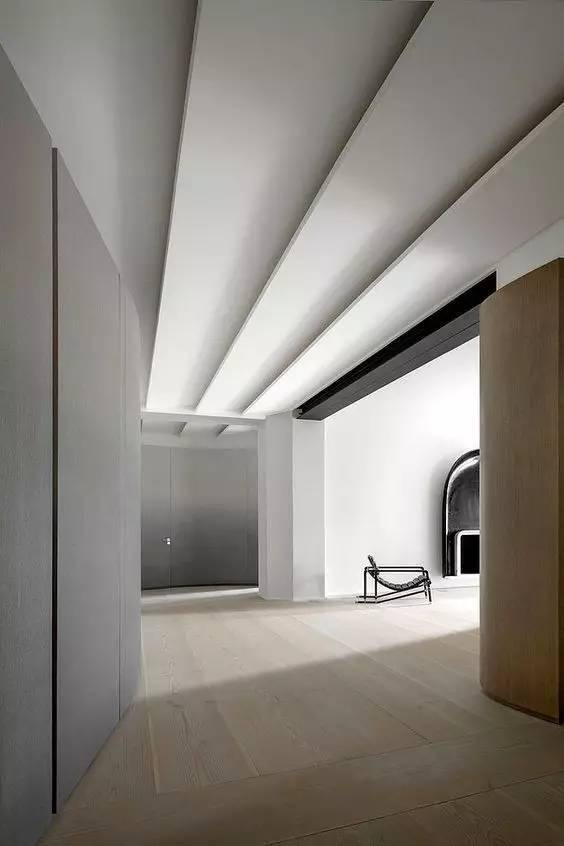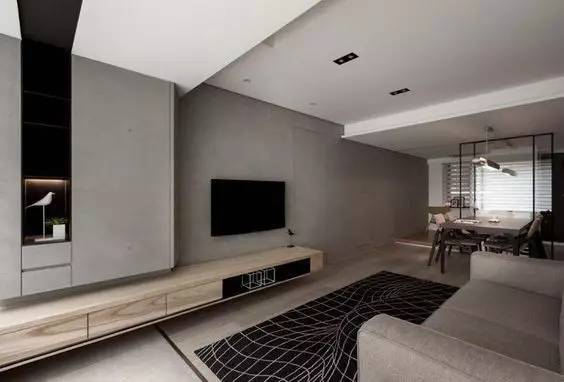材料选择上也非常多样，依据整体风格而定。金属、石膏、木板或玻璃材料，都可以用来做吊顶。总而言之，要不要做吊顶，做哪种形式的吊顶，要根据户型、层高、房顶结构、个人需求、预算等方面综合考虑。在现实生活中，不做吊顶的大有人在。牺牲层高而强行追求头顶那片“星空”的美观度，本身就是不可取的。如果觉得光秃秃的顶面过于单调，不妨对顶面进行简单的修饰和处理。事实上，那种不著痕迹的处理方法，更能带给人一种朴质无华、静谧高贵的感觉，因为它凸显了一种低调的魅力，与全球劲吹的简约之风正好不谋而合。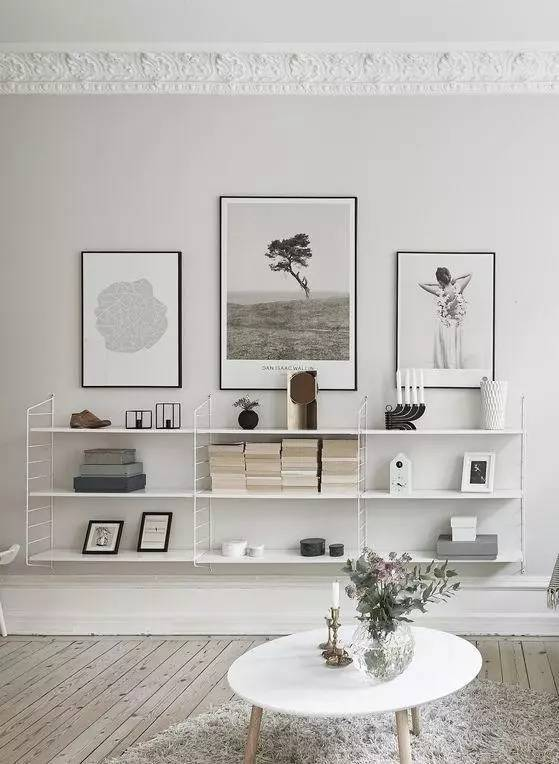编辑留言：

在了解了吊顶装修前需考虑的要点后，接下来就是和设计师沟通，确认吊顶的风格、色彩、款式等，然后由设计师出具图纸和完整的报价单，对实际装修起指导作用。

`声明：本文由入驻焦点开放平台的作者撰写，除焦点官方账号外，观点仅代表作者本人，不代表焦点立场错误信息举报电话： 400-099-0099，邮箱：jubao@vip.sohu.com，或点此进行意见反馈，或点此进行举报投诉。`A B C D E F G H J K L M N P Q R S T W X Y Z
A - B - C - D - E
• A
• 鞍山
• 安庆
• 安阳
• 安顺
• 安康
• 澳门
• B
• 北京
• 保定
• 包头
• 巴彦淖尔
• 本溪
• 蚌埠
• 亳州
• 滨州
• 北海
• 百色
• 巴中
• 毕节
• 保山
• 宝鸡
• 白银
• 巴州
• C
• 承德
• 沧州
• 长治
• 赤峰
• 朝阳
• 长春
• 常州
• 滁州
• 池州
• 长沙
• 常德
• 郴州
• 潮州
• 崇左
• 重庆
• 成都
• 楚雄
• 昌都
• 慈溪
• 常熟
• D
• 大同
• 大连
• 丹东
• 大庆
• 东营
• 德州
• 东莞
• 德阳
• 达州
• 大理
• 德宏
• 定西
• 儋州
• 东平
• E
• 鄂尔多斯
• 鄂州
• 恩施
F - G - H - I - J
• F
• 抚顺
• 阜新
• 阜阳
• 福州
• 抚州
• 佛山
• 防城港
• G
• 赣州
• 广州
• 桂林
• 贵港
• 广元
• 广安
• 贵阳
• 固原
• H
• 邯郸
• 衡水
• 呼和浩特
• 呼伦贝尔
• 葫芦岛
• 哈尔滨
• 黑河
• 淮安
• 杭州
• 湖州
• 合肥
• 淮南
• 淮北
• 黄山
• 菏泽
• 鹤壁
• 黄石
• 黄冈
• 衡阳
• 怀化
• 惠州
• 河源
• 贺州
• 河池
• 海口
• 红河
• 汉中
• 海东
• 怀来
• I
• J
• 晋中
• 锦州
• 吉林
• 鸡西
• 佳木斯
• 嘉兴
• 金华
• 景德镇
• 九江
• 吉安
• 济南
• 济宁
• 焦作
• 荆门
• 荆州
• 江门
• 揭阳
• 金昌
• 酒泉
• 嘉峪关
K - L - M - N - P
• K
• 开封
• 昆明
• 昆山
• L
• 廊坊
• 临汾
• 辽阳
• 连云港
• 丽水
• 六安
• 龙岩
• 莱芜
• 临沂
• 聊城
• 洛阳
• 漯河
• 娄底
• 柳州
• 来宾
• 泸州
• 乐山
• 六盘水
• 丽江
• 临沧
• 拉萨
• 林芝
• 兰州
• 陇南
• M
• 牡丹江
• 马鞍山
• 茂名
• 梅州
• 绵阳
• 眉山
• N
• 南京
• 南通
• 宁波
• 南平
• 宁德
• 南昌
• 南阳
• 南宁
• 内江
• 南充
• P
• 盘锦
• 莆田
• 平顶山
• 濮阳
• 攀枝花
• 普洱
• 平凉
Q - R - S - T - W
• Q
• 秦皇岛
• 齐齐哈尔
• 衢州
• 泉州
• 青岛
• 清远
• 钦州
• 黔南
• 曲靖
• 庆阳
• R
• 日照
• 日喀则
• S
• 石家庄
• 沈阳
• 双鸭山
• 绥化
• 上海
• 苏州
• 宿迁
• 绍兴
• 宿州
• 三明
• 上饶
• 三门峡
• 商丘
• 十堰
• 随州
• 邵阳
• 韶关
• 深圳
• 汕头
• 汕尾
• 三亚
• 三沙
• 遂宁
• 山南
• 商洛
• 石嘴山
• T
• 天津
• 唐山
• 太原
• 通辽
• 铁岭
• 泰州
• 台州
• 铜陵
• 泰安
• 铜仁
• 铜川
• 天水
• 天门
• W
• 乌海
• 乌兰察布
• 无锡
• 温州
• 芜湖
• 潍坊
• 威海
• 武汉
• 梧州
• 渭南
• 武威
• 吴忠
• 乌鲁木齐
X - Y - Z
• X
• 邢台
• 徐州
• 宣城
• 厦门
• 新乡
• 许昌
• 信阳
• 襄阳
• 孝感
• 咸宁
• 湘潭
• 湘西
• 西双版纳
• 西安
• 咸阳
• 西宁
• 仙桃
• 西昌
• Y
• 运城
• 营口
• 盐城
• 扬州
• 鹰潭
• 宜春
• 烟台
• 宜昌
• 岳阳
• 益阳
• 永州
• 阳江
• 云浮
• 玉林
• 宜宾
• 雅安
• 玉溪
• 延安
• 榆林
• 银川
• Z
• 张家口
• 镇江
• 舟山
• 漳州
• 淄博
• 枣庄
• 郑州
• 周口
• 驻马店
• 株洲
• 张家界
• 珠海
• 湛江
• 肇庆
• 中山
• 自贡
• 资阳
• 遵义
• 昭通
• 张掖
• 中卫

1室1厅1厨1卫1阳台

1
2
3
4
5

0
1
2

1

1

0
1
2
3报名成功，资料已提交审核A B C D E F G H J K L M N P Q R S T W X Y Z
A - B - C - D - E
• A
• 鞍山
• 安庆
• 安阳
• 安顺
• 安康
• 澳门
• B
• 北京
• 保定
• 包头
• 巴彦淖尔
• 本溪
• 蚌埠
• 亳州
• 滨州
• 北海
• 百色
• 巴中
• 毕节
• 保山
• 宝鸡
• 白银
• 巴州
• C
• 承德
• 沧州
• 长治
• 赤峰
• 朝阳
• 长春
• 常州
• 滁州
• 池州
• 长沙
• 常德
• 郴州
• 潮州
• 崇左
• 重庆
• 成都
• 楚雄
• 昌都
• 慈溪
• 常熟
• D
• 大同
• 大连
• 丹东
• 大庆
• 东营
• 德州
• 东莞
• 德阳
• 达州
• 大理
• 德宏
• 定西
• 儋州
• 东平
• E
• 鄂尔多斯
• 鄂州
• 恩施
F - G - H - I - J
• F
• 抚顺
• 阜新
• 阜阳
• 福州
• 抚州
• 佛山
• 防城港
• G
• 赣州
• 广州
• 桂林
• 贵港
• 广元
• 广安
• 贵阳
• 固原
• H
• 邯郸
• 衡水
• 呼和浩特
• 呼伦贝尔
• 葫芦岛
• 哈尔滨
• 黑河
• 淮安
• 杭州
• 湖州
• 合肥
• 淮南
• 淮北
• 黄山
• 菏泽
• 鹤壁
• 黄石
• 黄冈
• 衡阳
• 怀化
• 惠州
• 河源
• 贺州
• 河池
• 海口
• 红河
• 汉中
• 海东
• 怀来
• I
• J
• 晋中
• 锦州
• 吉林
• 鸡西
• 佳木斯
• 嘉兴
• 金华
• 景德镇
• 九江
• 吉安
• 济南
• 济宁
• 焦作
• 荆门
• 荆州
• 江门
• 揭阳
• 金昌
• 酒泉
• 嘉峪关
K - L - M - N - P
• K
• 开封
• 昆明
• 昆山
• L
• 廊坊
• 临汾
• 辽阳
• 连云港
• 丽水
• 六安
• 龙岩
• 莱芜
• 临沂
• 聊城
• 洛阳
• 漯河
• 娄底
• 柳州
• 来宾
• 泸州
• 乐山
• 六盘水
• 丽江
• 临沧
• 拉萨
• 林芝
• 兰州
• 陇南
• M
• 牡丹江
• 马鞍山
• 茂名
• 梅州
• 绵阳
• 眉山
• N
• 南京
• 南通
• 宁波
• 南平
• 宁德
• 南昌
• 南阳
• 南宁
• 内江
• 南充
• P
• 盘锦
• 莆田
• 平顶山
• 濮阳
• 攀枝花
• 普洱
• 平凉
Q - R - S - T - W
• Q
• 秦皇岛
• 齐齐哈尔
• 衢州
• 泉州
• 青岛
• 清远
• 钦州
• 黔南
• 曲靖
• 庆阳
• R
• 日照
• 日喀则
• S
• 石家庄
• 沈阳
• 双鸭山
• 绥化
• 上海
• 苏州
• 宿迁
• 绍兴
• 宿州
• 三明
• 上饶
• 三门峡
• 商丘
• 十堰
• 随州
• 邵阳
• 韶关
• 深圳
• 汕头
• 汕尾
• 三亚
• 三沙
• 遂宁
• 山南
• 商洛
• 石嘴山
• T
• 天津
• 唐山
• 太原
• 通辽
• 铁岭
• 泰州
• 台州
• 铜陵
• 泰安
• 铜仁
• 铜川
• 天水
• 天门
• W
• 乌海
• 乌兰察布
• 无锡
• 温州
• 芜湖
• 潍坊
• 威海
• 武汉
• 梧州
• 渭南
• 武威
• 吴忠
• 乌鲁木齐
X - Y - Z
• X
• 邢台
• 徐州
• 宣城
• 厦门
• 新乡
• 许昌
• 信阳
• 襄阳
• 孝感
• 咸宁
• 湘潭
• 湘西
• 西双版纳
• 西安
• 咸阳
• 西宁
• 仙桃
• 西昌
• Y
• 运城
• 营口
• 盐城
• 扬州
• 鹰潭
• 宜春
• 烟台
• 宜昌
• 岳阳
• 益阳
• 永州
• 阳江
• 云浮
• 玉林
• 宜宾
• 雅安
• 玉溪
• 延安
• 榆林
• 银川
• Z
• 张家口
• 镇江
• 舟山
• 漳州
• 淄博
• 枣庄
• 郑州
• 周口
• 驻马店
• 株洲
• 张家界
• 珠海
• 湛江
• 肇庆
• 中山
• 自贡
• 资阳
• 遵义
• 昭通
• 张掖
• 中卫• 手机• 分享
• 设计
免费设计
• 计算器
装修计算器
• 入驻
合作入驻
• 联系
联系我们
• 置顶
返回顶部